# Bar Le Bar de la programmation

## Recommended Posts

miiince j'avais pas vu ton msg dsl sa a changé de page

• Replies 745
• Created

#### Popular Days

sa veut pas ce compiler et s'exécuter !!!

```#include <stdio.h>
#include <stdlib.h>
#include <time.h>

long genereHaz()
{
long nb = 0;
const long MAX = 36, MIN = 0;
srand(time(NULL));
nb = (rand() % (MAX - MIN + 1)) + MIN;
return nb;
}

int main(int argc, char *argv[])
{
long serie = 0;
printf("Generateur de series aleatoire");
printf("\n\t\t\tby K-1!BR3");
printf("\n\nCombien de nombres aleatoire veus-tu ?\n");
scanf("%ld", &serie);
long compteur = 0;
printf("\n");
for (compteur = 0 ; compteur < serie ; compteur++)
{

long nb = genereHaz();
if (nb == 0)
{
printf("\n0  Vert");
FILE* fichier = NULL;
fichier = fopen("Generateur de series/serie.txt", "a+");
if (fichier != NULL)
{
fputs("\n0  Vert", fichier);
fclose(fichier);
}
else
printf(_strerror(NULL));

}

else if (nb == 1)
{
printf("\n0  Vert");
FILE* fichier = NULL;
fichier = fopen("Generateur de series/serie.txt", "a+");
if (fichier != NULL)
{
fputs("\n0  Vert", fichier);
fclose(fichier);
}
else
printf(_strerror(NULL));

}

else if (nb == 2)
{
printf("\n0  Vert");
FILE* fichier = NULL;
fichier = fopen("Generateur de series/serie.txt", "a+");
if (fichier != NULL)
{
fputs("\n0  Vert", fichier);
fclose(fichier);
}
else
printf(_strerror(NULL));

}

else if (nb == 3)
{
printf("\n0  Vert");
FILE* fichier = NULL;
fichier = fopen("Generateur de series/serie.txt", "a+");
if (fichier != NULL)
{
fputs("\n0  Vert", fichier);
fclose(fichier);
}
else
printf(_strerror(NULL));
}

else if (nb == 4)
{
printf("\n0  Vert");
FILE* fichier = NULL;
fichier = fopen("Generateur de series/serie.txt", "a+");
if (fichier != NULL)
{
fputs("\n0  Vert", fichier);
fclose(fichier);
}
else
printf(_strerror(NULL));
}

else if (nb == 5)
{
printf("\n0  Vert");
FILE* fichier = NULL;
fichier = fopen("Generateur de series/serie.txt", "a+");
if (fichier != NULL)
{
fputs("\n0  Vert", fichier);
fclose(fichier);
}
else
printf(_strerror(NULL));
}

else if (nb == 6)
{
printf("\n0  Vert");
FILE* fichier = NULL;
fichier = fopen("Generateur de series/serie.txt", "a+");
if (fichier != NULL)
{
fputs("\n0  Vert", fichier);
fclose(fichier);
}
else
printf(_strerror(NULL));
}

else if (nb == 7)
{
printf("\n0  Vert");
FILE* fichier = NULL;
fichier = fopen("Generateur de series/serie.txt", "a+");
if (fichier != NULL)
{
fputs("\n0  Vert", fichier);
fclose(fichier);
}
else
printf(_strerror(NULL));
}

else if (nb ==
{
printf("\n0  Vert");
FILE* fichier = NULL;
fichier = fopen("Generateur de series/serie.txt", "a+");
if (fichier != NULL)
{
fputs("\n0  Vert", fichier);
fclose(fichier);
}
else
printf(_strerror(NULL));
}

else if (nb == 9)
{
printf("\n0  Vert");
FILE* fichier = NULL;
fichier = fopen("Generateur de series/serie.txt", "a+");
if (fichier != NULL)
{
fputs("\n0  Vert", fichier);
fclose(fichier);
}
else
printf(_strerror(NULL));
}

else if (nb == 10)
{
printf("\n0  Vert");
FILE* fichier = NULL;
fichier = fopen("Generateur de series/serie.txt", "a+");
if (fichier != NULL)
{
fputs("\n0  Vert", fichier);
fclose(fichier);
}
else
printf(_strerror(NULL));
}

else if (nb == 11)
{
printf("\n0  Vert");
FILE* fichier = NULL;
fichier = fopen("Generateur de series/serie.txt", "a+");
if (fichier != NULL)
{
fputs("\n0  Vert", fichier);
fclose(fichier);
}
else
printf(_strerror(NULL));
}

else if (nb == 12)
{
printf("\n0  Vert");
FILE* fichier = NULL;
fichier = fopen("Generateur de series/serie.txt", "a+");
if (fichier != NULL)
{
fputs("\n0  Vert", fichier);
fclose(fichier);
}
else
printf(_strerror(NULL));
}

else if (nb == 13)
{
printf("\n0  Vert");
FILE* fichier = NULL;
fichier = fopen("Generateur de series/serie.txt", "a+");
if (fichier != NULL)
{
fputs("\n0  Vert", fichier);
fclose(fichier);
}
else
printf(_strerror(NULL));
}

else if (nb == 14)
{
printf("\n0  Vert");
FILE* fichier = NULL;
fichier = fopen("Generateur de series/serie.txt", "a+");
if (fichier != NULL)
{
fputs("\n0  Vert", fichier);
fclose(fichier);
}
else
printf(_strerror(NULL));
}

else if (nb == 15)
{
printf("\n0  Vert");
FILE* fichier = NULL;
fichier = fopen("Generateur de series/serie.txt", "a+");
if (fichier != NULL)
{
fputs("\n0  Vert", fichier);
fclose(fichier);
}
else
printf(_strerror(NULL));
}

else if (nb == 16)
{
printf("\n0  Vert");
FILE* fichier = NULL;
fichier = fopen("Generateur de series/serie.txt", "a+");
if (fichier != NULL)
{
fputs("\n0  Vert", fichier);
fclose(fichier);
}
else
printf(_strerror(NULL));
}

else if (nb == 16)
{
printf("\n0  Vert");
FILE* fichier = NULL;
fichier = fopen("Generateur de series/serie.txt", "a+");
if (fichier != NULL)
{
fputs("\n0  Vert", fichier);
fclose(fichier);
}
else
printf(_strerror(NULL));
}

else if (nb == 17)
{
printf("\n0  Vert");
FILE* fichier = NULL;
fichier = fopen("Generateur de series/serie.txt", "a+");
if (fichier != NULL)
{
fputs("\n0  Vert", fichier);
fclose(fichier);
}
else
printf(_strerror(NULL));
}

else if (nb == 18)
{
printf("\n0  Vert");
FILE* fichier = NULL;
fichier = fopen("Generateur de series/serie.txt", "a+");
if (fichier != NULL)
{
fputs("\n0  Vert", fichier);
fclose(fichier);
}
else
printf(_strerror(NULL));
}

else if (nb == 19)
{
printf("\n0  Vert");
FILE* fichier = NULL;
fichier = fopen("Generateur de series/serie.txt", "a+");
if (fichier != NULL)
{
fputs("\n0  Vert", fichier);
fclose(fichier);
}
else
printf(_strerror(NULL));
}

else if (nb == 20)
{
printf("\n0  Vert");
FILE* fichier = NULL;
fichier = fopen("Generateur de series/serie.txt", "a+");
if (fichier != NULL)
{
fputs("\n0  Vert", fichier);
fclose(fichier);
}
else
printf(_strerror(NULL));
}

else if (nb == 21)
{
printf("\n0  Vert");
FILE* fichier = NULL;
fichier = fopen("Generateur de series/serie.txt", "a+");
if (fichier != NULL)
{
fputs("\n0  Vert", fichier);
fclose(fichier);
}
else
printf(_strerror(NULL));
}

else if (nb == 22)
{
printf("\n0  Vert");
FILE* fichier = NULL;
fichier = fopen("Generateur de series/serie.txt", "a+");
if (fichier != NULL)
{
fputs("\n0  Vert", fichier);
fclose(fichier);
}
else
printf(_strerror(NULL));
}

else if (nb == 23)
{
printf("\n0  Vert");
FILE* fichier = NULL;
fichier = fopen("Generateur de series/serie.txt", "a+");
if (fichier != NULL)
{
fputs("\n0  Vert", fichier);
fclose(fichier);
}
else
printf(_strerror(NULL));
}

else if (nb == 24)
{
printf("\n0  Vert");
FILE* fichier = NULL;
fichier = fopen("Generateur de series/serie.txt", "a+");
if (fichier != NULL)
{
fputs("\n0  Vert", fichier);
fclose(fichier);
}
else
printf(_strerror(NULL));
}

else if (nb == 25)
{
printf("\n0  Vert");
FILE* fichier = NULL;
fichier = fopen("Generateur de series/serie.txt", "a+");
if (fichier != NULL)
{
fputs("\n0  Vert", fichier);
fclose(fichier);
}
else
printf(_strerror(NULL));
}

else if (nb == 26)
{
printf("\n0  Vert");
FILE* fichier = NULL;
fichier = fopen("Generateur de series/serie.txt", "a+");
if (fichier != NULL)
{
fputs("\n0  Vert", fichier);
fclose(fichier);
}
else
{
printf(_strerror(NULL));
}

}

else if (nb == 27)
{
printf("\n0  Vert");
FILE* fichier = NULL;
fichier = fopen("Generateur de series/serie.txt", "a+");
if (fichier != NULL)
{
fputs("\n0  Vert", fichier);
fclose(fichier);
}
else
printf(_strerror(NULL));
}

else if (nb == 28)
{
printf("\n0  Vert");
FILE* fichier = NULL;
fichier = fopen("Generateur de series/serie.txt", "a+");
if (fichier != NULL)
{
fputs("\n0  Vert", fichier);
fclose(fichier);
}
else
printf(_strerror(NULL));
}

else if (nb == 29)
{
printf("\n0  Vert");
FILE* fichier = NULL;
fichier = fopen("Generateur de series/serie.txt", "a+");
if (fichier != NULL)
{
fputs("\n0  Vert", fichier);
fclose(fichier);
}
else
printf(_strerror(NULL));
}

else if (nb == 30)
{
printf("\n0  Vert");
FILE* fichier = NULL;
fichier = fopen("Generateur de series/serie.txt", "a+");
if (fichier != NULL)
{
fputs("\n0  Vert", fichier);
fclose(fichier);
}
else
printf(_strerror(NULL));
}

else if (nb == 31)
{
printf("\n0  Vert");
FILE* fichier = NULL;
fichier = fopen("Generateur de series/serie.txt", "a+");
if (fichier != NULL)
{
fputs("\n0  Vert", fichier);
fclose(fichier);
}
else
printf(_strerror(NULL));
}

else if (nb == 32)
{
printf("\n0  Vert");
FILE* fichier = NULL;
fichier = fopen("Generateur de series/serie.txt", "a+");
if (fichier != NULL)
{
fputs("\n0  Vert", fichier);
fclose(fichier);
}
else
printf(_strerror(NULL));
}

else if (nb == 33)
{
printf("\n0  Vert");
FILE* fichier = NULL;
fichier = fopen("Generateur de series/serie.txt", "a+");
if (fichier != NULL)
{
fputs("\n0  Vert", fichier);
fclose(fichier);
}
else
printf(_strerror(NULL));
}

else if (nb == 34)
{
printf("\n0  Vert");
FILE* fichier = NULL;
fichier = fopen("Generateur de series/serie.txt", "a+");
if (fichier != NULL)
{
fputs("\n0  Vert", fichier);
fclose(fichier);
}
else
printf(_strerror(NULL));
}

else if (nb == 35)
{
printf("\n0  Vert");
FILE* fichier = NULL;
fichier = fopen("Generateur de series/serie.txt", "a+");
if (fichier != NULL)
{
fputs("\n0  Vert", fichier);
fclose(fichier);
}
else
printf(_strerror(NULL));
}

else if (nb == 36)
{
printf("\n0  Vert");
FILE* fichier = NULL;
fichier = fopen("Generateur de series/serie.txt", "a+");
if (fichier != NULL)
{
fputs("\n0  Vert", fichier);
fclose(fichier);
}
else
printf(_strerror(NULL));
}

}

printf("\nUn bloc note contenant la serie a ete cree dans le dossier Generateur de series\n\n");
system("PAUSE");
return 0;
}
```

38 C:\Documents and Settings\user\Bureau\Generateur de series\mainSerie.c [Warning] passing arg 1 of `printf' makes pointer from integer without a cast

##### Share on other sites

Salut,

Déjà tu peux voir quel est le problème en affichant le pourquoi de l'erreur. Par exemple :

```if (fichier != NULL)
{
// ...
}
else
{
printf(_strerror(NULL)); // Affiche le texte de description de la dernière erreur rencontrée par le programme
}```

printf(_strerror(NULL)); ne fonctionnera jamais. Peut être

`printf("%s",_strerror(NULL));`

Je ne suis pas sur que tu puisses utiliser _strerror() avec Dev C++.

Et il faut créer le répertoire "Generateur de series" ou qu'il existe dans le répertoire d'exécution de ton application.

Cordialement,

Barroud.

##### Share on other sites

merci sa marche voici l'erreur :

no such file or directory

##### Share on other sites

Ton code fonctionne chez moi avec Dev C++ 4.9.9.2. Il suffit juste de créer le répertoire "Generateur de series" dans le répertoire d'exécution de ton logiciel.

##### Share on other sites

ah mais ouiiiiiiiiiiiiiiiiiiiii, j'ai fais pour le répertoire d'au dessus moi xD

voila pour ceux que sa intéresse :

```#include <stdio.h>
#include <stdlib.h>
#include <time.h>

long genereHaz()
{
long nb = 0;
const long MAX = 36, MIN = 0;
srand(time(NULL));
nb = (rand() % (MAX - MIN + 1)) + MIN;
return nb;
}

int main(int argc, char *argv[])
{
long serie = 0;
printf("Generateur de series aleatoire");
printf("\n\t\t\tby K-1!BR3");
printf("\n\nCombien de nombres aleatoire voulez-vous ?\n");
scanf("%ld", &serie);
long compteur = 0;
printf("\n");
for (compteur = 0 ; compteur < serie ; compteur++)
{

long nb = genereHaz();
if (nb == 0)
{
printf("\n0  Vert");
FILE* fichier = NULL;
fichier = fopen("Generateur de series/serie.txt", "a+");
if (fichier != NULL)
{
fputs("\n0  Vert", fichier);
fclose(fichier);
}
else
printf("%s",_strerror(NULL));

}

else if (nb == 1)
{
printf("\n0  Vert");
FILE* fichier = NULL;
fichier = fopen("Generateur de series/serie.txt", "a+");
if (fichier != NULL)
{
fputs("\n0  Vert", fichier);
fclose(fichier);
}
else
printf("%s",_strerror(NULL));

}

else if (nb == 2)
{
printf("\n0  Vert");
FILE* fichier = NULL;
fichier = fopen("Generateur de series/serie.txt", "a+");
if (fichier != NULL)
{
fputs("\n0  Vert", fichier);
fclose(fichier);
}
else
printf("%s",_strerror(NULL));

}

else if (nb == 3)
{
printf("\n0  Vert");
FILE* fichier = NULL;
fichier = fopen("Generateur de series/serie.txt", "a+");
if (fichier != NULL)
{
fputs("\n0  Vert", fichier);
fclose(fichier);
}
else
printf("%s",_strerror(NULL));
}

else if (nb == 4)
{
printf("\n0  Vert");
FILE* fichier = NULL;
fichier = fopen("Generateur de series/serie.txt", "a+");
if (fichier != NULL)
{
fputs("\n0  Vert", fichier);
fclose(fichier);
}
else
printf("%s",_strerror(NULL));
}

else if (nb == 5)
{
printf("\n0  Vert");
FILE* fichier = NULL;
fichier = fopen("Generateur de series/serie.txt", "a+");
if (fichier != NULL)
{
fputs("\n0  Vert", fichier);
fclose(fichier);
}
else
printf("%s",_strerror(NULL));
}

else if (nb == 6)
{
printf("\n0  Vert");
FILE* fichier = NULL;
fichier = fopen("Generateur de series/serie.txt", "a+");
if (fichier != NULL)
{
fputs("\n0  Vert", fichier);
fclose(fichier);
}
else
printf("%s",_strerror(NULL));
}

else if (nb == 7)
{
printf("\n0  Vert");
FILE* fichier = NULL;
fichier = fopen("Generateur de series/serie.txt", "a+");
if (fichier != NULL)
{
fputs("\n0  Vert", fichier);
fclose(fichier);
}
else
printf("%s",_strerror(NULL));
}

else if (nb ==
{
printf("\n0  Vert");
FILE* fichier = NULL;
fichier = fopen("Generateur de series/serie.txt", "a+");
if (fichier != NULL)
{
fputs("\n0  Vert", fichier);
fclose(fichier);
}
else
printf("%s",_strerror(NULL));
}

else if (nb == 9)
{
printf("\n0  Vert");
FILE* fichier = NULL;
fichier = fopen("Generateur de series/serie.txt", "a+");
if (fichier != NULL)
{
fputs("\n0  Vert", fichier);
fclose(fichier);
}
else
printf("%s",_strerror(NULL));
}

else if (nb == 10)
{
printf("\n0  Vert");
FILE* fichier = NULL;
fichier = fopen("Generateur de series/serie.txt", "a+");
if (fichier != NULL)
{
fputs("\n0  Vert", fichier);
fclose(fichier);
}
else
printf("%s",_strerror(NULL));
}

else if (nb == 11)
{
printf("\n0  Vert");
FILE* fichier = NULL;
fichier = fopen("Generateur de series/serie.txt", "a+");
if (fichier != NULL)
{
fputs("\n0  Vert", fichier);
fclose(fichier);
}
else
printf("%s",_strerror(NULL));
}

else if (nb == 12)
{
printf("\n0  Vert");
FILE* fichier = NULL;
fichier = fopen("Generateur de series/serie.txt", "a+");
if (fichier != NULL)
{
fputs("\n0  Vert", fichier);
fclose(fichier);
}
else
printf("%s",_strerror(NULL));
}

else if (nb == 13)
{
printf("\n0  Vert");
FILE* fichier = NULL;
fichier = fopen("Generateur de series/serie.txt", "a+");
if (fichier != NULL)
{
fputs("\n0  Vert", fichier);
fclose(fichier);
}
else
printf("%s",_strerror(NULL));
}

else if (nb == 14)
{
printf("\n0  Vert");
FILE* fichier = NULL;
fichier = fopen("Generateur de series/serie.txt", "a+");
if (fichier != NULL)
{
fputs("\n0  Vert", fichier);
fclose(fichier);
}
else
printf("%s",_strerror(NULL));
}

else if (nb == 15)
{
printf("\n0  Vert");
FILE* fichier = NULL;
fichier = fopen("Generateur de series/serie.txt", "a+");
if (fichier != NULL)
{
fputs("\n0  Vert", fichier);
fclose(fichier);
}
else
printf("%s",_strerror(NULL));
}

else if (nb == 16)
{
printf("\n0  Vert");
FILE* fichier = NULL;
fichier = fopen("Generateur de series/serie.txt", "a+");
if (fichier != NULL)
{
fputs("\n0  Vert", fichier);
fclose(fichier);
}
else
printf("%s",_strerror(NULL));
}

else if (nb == 16)
{
printf("\n0  Vert");
FILE* fichier = NULL;
fichier = fopen("Generateur de series/serie.txt", "a+");
if (fichier != NULL)
{
fputs("\n0  Vert", fichier);
fclose(fichier);
}
else
printf("%s",_strerror(NULL));
}

else if (nb == 17)
{
printf("\n0  Vert");
FILE* fichier = NULL;
fichier = fopen("Generateur de series/serie.txt", "a+");
if (fichier != NULL)
{
fputs("\n0  Vert", fichier);
fclose(fichier);
}
else
printf("%s",_strerror(NULL));
}

else if (nb == 18)
{
printf("\n0  Vert");
FILE* fichier = NULL;
fichier = fopen("Generateur de series/serie.txt", "a+");
if (fichier != NULL)
{
fputs("\n0  Vert", fichier);
fclose(fichier);
}
else
printf("%s",_strerror(NULL));
}

else if (nb == 19)
{
printf("\n0  Vert");
FILE* fichier = NULL;
fichier = fopen("Generateur de series/serie.txt", "a+");
if (fichier != NULL)
{
fputs("\n0  Vert", fichier);
fclose(fichier);
}
else
printf("%s",_strerror(NULL));
}

else if (nb == 20)
{
printf("\n0  Vert");
FILE* fichier = NULL;
fichier = fopen("Generateur de series/serie.txt", "a+");
if (fichier != NULL)
{
fputs("\n0  Vert", fichier);
fclose(fichier);
}
else
printf("%s",_strerror(NULL));
}

else if (nb == 21)
{
printf("\n0  Vert");
FILE* fichier = NULL;
fichier = fopen("Generateur de series/serie.txt", "a+");
if (fichier != NULL)
{
fputs("\n0  Vert", fichier);
fclose(fichier);
}
else
printf("%s",_strerror(NULL));
}

else if (nb == 22)
{
printf("\n0  Vert");
FILE* fichier = NULL;
fichier = fopen("Generateur de series/serie.txt", "a+");
if (fichier != NULL)
{
fputs("\n0  Vert", fichier);
fclose(fichier);
}
else
printf("%s",_strerror(NULL));
}

else if (nb == 23)
{
printf("\n0  Vert");
FILE* fichier = NULL;
fichier = fopen("Generateur de series/serie.txt", "a+");
if (fichier != NULL)
{
fputs("\n0  Vert", fichier);
fclose(fichier);
}
else
printf("%s",_strerror(NULL));
}

else if (nb == 24)
{
printf("\n0  Vert");
FILE* fichier = NULL;
fichier = fopen("Generateur de series/serie.txt", "a+");
if (fichier != NULL)
{
fputs("\n0  Vert", fichier);
fclose(fichier);
}
else
printf("%s",_strerror(NULL));
}

else if (nb == 25)
{
printf("\n0  Vert");
FILE* fichier = NULL;
fichier = fopen("Generateur de series/serie.txt", "a+");
if (fichier != NULL)
{
fputs("\n0  Vert", fichier);
fclose(fichier);
}
else
printf("%s",_strerror(NULL));
}

else if (nb == 26)
{
printf("\n0  Vert");
FILE* fichier = NULL;
fichier = fopen("Generateur de series/serie.txt", "a+");
if (fichier != NULL)
{
fputs("\n0  Vert", fichier);
fclose(fichier);
}
else
{
printf("%s",_strerror(NULL));
}

}

else if (nb == 27)
{
printf("\n0  Vert");
FILE* fichier = NULL;
fichier = fopen("Generateur de series/serie.txt", "a+");
if (fichier != NULL)
{
fputs("\n0  Vert", fichier);
fclose(fichier);
}
else
printf("%s",_strerror(NULL));
}

else if (nb == 28)
{
printf("\n0  Vert");
FILE* fichier = NULL;
fichier = fopen("Generateur de series/serie.txt", "a+");
if (fichier != NULL)
{
fputs("\n0  Vert", fichier);
fclose(fichier);
}
else
printf("%s",_strerror(NULL));
}

else if (nb == 29)
{
printf("\n0  Vert");
FILE* fichier = NULL;
fichier = fopen("Generateur de series/serie.txt", "a+");
if (fichier != NULL)
{
fputs("\n0  Vert", fichier);
fclose(fichier);
}
else
printf("%s",_strerror(NULL));
}

else if (nb == 30)
{
printf("\n0  Vert");
FILE* fichier = NULL;
fichier = fopen("Generateur de series/serie.txt", "a+");
if (fichier != NULL)
{
fputs("\n0  Vert", fichier);
fclose(fichier);
}
else
printf("%s",_strerror(NULL));
}

else if (nb == 31)
{
printf("\n0  Vert");
FILE* fichier = NULL;
fichier = fopen("Generateur de series/serie.txt", "a+");
if (fichier != NULL)
{
fputs("\n0  Vert", fichier);
fclose(fichier);
}
else
printf("%s",_strerror(NULL));
}

else if (nb == 32)
{
printf("\n0  Vert");
FILE* fichier = NULL;
fichier = fopen("Generateur de series/serie.txt", "a+");
if (fichier != NULL)
{
fputs("\n0  Vert", fichier);
fclose(fichier);
}
else
printf("%s",_strerror(NULL));
}

else if (nb == 33)
{
printf("\n0  Vert");
FILE* fichier = NULL;
fichier = fopen("Generateur de series/serie.txt", "a+");
if (fichier != NULL)
{
fputs("\n0  Vert", fichier);
fclose(fichier);
}
else
printf("%s",_strerror(NULL));
}

else if (nb == 34)
{
printf("\n0  Vert");
FILE* fichier = NULL;
fichier = fopen("Generateur de series/serie.txt", "a+");
if (fichier != NULL)
{
fputs("\n0  Vert", fichier);
fclose(fichier);
}
else
printf("%s",_strerror(NULL));
}

else if (nb == 35)
{
printf("\n0  Vert");
FILE* fichier = NULL;
fichier = fopen("Generateur de series/serie.txt", "a+");
if (fichier != NULL)
{
fputs("\n0  Vert", fichier);
fclose(fichier);
}
else
printf("%s",_strerror(NULL));
}

else if (nb == 36)
{
printf("\n0  Vert");
FILE* fichier = NULL;
fichier = fopen("Generateur de series/serie.txt", "a+");
if (fichier != NULL)
{
fputs("\n0  Vert", fichier);
fclose(fichier);
}
else
printf("%s",_strerror(NULL));
}

}

printf("\n\n\nUn bloc note contenant la serie a ete cree dans le dossier Generateur de series\n\nSi une erreur s'est produite, vous devez cree un dossier Generateur de series dans le meme dossier que l'application.\n\n");
system("PAUSE");
return 0;
}
```

il manque plus qu'a remplacer les 0 vert par les différent numéro, couleur, impair ,passe .... je vous donne ça quand je l'aurais fais

merci à tous

##### Share on other sites

j'ai finis mais il y a encore un problème, le logiciel me donne le meme nombre à chaque retour de la boucle for c'est bizarre :S

genereHaz renvoit bien un nombre au hazard mais le meme à chaque "for"

```#include <stdio.h>
#include <stdlib.h>
#include <time.h>

long genereHaz()
{
long nb = 0;
const long MAX = 36, MIN = 0;
srand(time(NULL));
nb = (rand() % (MAX - MIN + 1)) + MIN;
return nb;
}

int main(int argc, char *argv[])
{
long serie = 0;
printf("Generateur de series aleatoire");
printf("\n\t\t\tby K-1!BR3");
printf("\n\nCombien de nombres aleatoire voulez-vous ?\n");
scanf("%ld", &serie);
long compteur = 0;
printf("\n");
long nb = 0;
for (compteur = 0 ; compteur < serie ; compteur++)
{

nb = genereHaz();
if (nb == 0)
{
printf("\n0   Vert");
FILE* fichier = NULL;
fichier = fopen("Generateur de series/serie.txt", "a+");
if (fichier != NULL)
{
fputs("\n0  Vert", fichier);
fclose(fichier);
}
else
printf("%s",_strerror(NULL));

}

else if (nb == 1)
{
printf("\n1   Rouge Impaire Manque");
FILE* fichier = NULL;
fichier = fopen("Generateur de series/serie.txt", "a+");
if (fichier != NULL)
{
fputs("\n0  Vert", fichier);
fclose(fichier);
}
else
printf("%s",_strerror(NULL));

}

else if (nb == 2)
{
printf("\n2   Noir  Paire   Manque");
FILE* fichier = NULL;
fichier = fopen("Generateur de series/serie.txt", "a+");
if (fichier != NULL)
{
fputs("\n0  Vert", fichier);
fclose(fichier);
}
else
printf("%s",_strerror(NULL));

}

else if (nb == 3)
{
printf("\n3   Rouge Impaire Manque");
FILE* fichier = NULL;
fichier = fopen("Generateur de series/serie.txt", "a+");
if (fichier != NULL)
{
fputs("\n0  Vert", fichier);
fclose(fichier);
}
else
printf("%s",_strerror(NULL));
}

else if (nb == 4)
{
printf("\n4   Noir  Paire   Manque");
FILE* fichier = NULL;
fichier = fopen("Generateur de series/serie.txt", "a+");
if (fichier != NULL)
{
fputs("\n0  Vert", fichier);
fclose(fichier);
}
else
printf("%s",_strerror(NULL));
}

else if (nb == 5)
{
printf("\n5   Rouge Impaire Manque");
FILE* fichier = NULL;
fichier = fopen("Generateur de series/serie.txt", "a+");
if (fichier != NULL)
{
fputs("\n0  Vert", fichier);
fclose(fichier);
}
else
printf("%s",_strerror(NULL));
}

else if (nb == 6)
{
printf("\n6   Noir  Paire   Manque");
FILE* fichier = NULL;
fichier = fopen("Generateur de series/serie.txt", "a+");
if (fichier != NULL)
{
fputs("\n0  Vert", fichier);
fclose(fichier);
}
else
printf("%s",_strerror(NULL));
}

else if (nb == 7)
{
printf("\n7   Rouge Impaire Manque");
FILE* fichier = NULL;
fichier = fopen("Generateur de series/serie.txt", "a+");
if (fichier != NULL)
{
fputs("\n0  Vert", fichier);
fclose(fichier);
}
else
printf("%s",_strerror(NULL));
}

else if (nb ==
{
printf("\n8   Noir  Paire   Manque");
FILE* fichier = NULL;
fichier = fopen("Generateur de series/serie.txt", "a+");
if (fichier != NULL)
{
fputs("\n0  Vert", fichier);
fclose(fichier);
}
else
printf("%s",_strerror(NULL));
}

else if (nb == 9)
{
printf("\n9   Rouge Impaire Manque");
FILE* fichier = NULL;
fichier = fopen("Generateur de series/serie.txt", "a+");
if (fichier != NULL)
{
fputs("\n0  Vert", fichier);
fclose(fichier);
}
else
printf("%s",_strerror(NULL));
}

else if (nb == 10)
{
printf("\n10  Noir  Paire   Manque");
FILE* fichier = NULL;
fichier = fopen("Generateur de series/serie.txt", "a+");
if (fichier != NULL)
{
fputs("\n0  Vert", fichier);
fclose(fichier);
}
else
printf("%s",_strerror(NULL));
}

else if (nb == 11)
{
printf("\n11  Noir  Impaire Manque");
FILE* fichier = NULL;
fichier = fopen("Generateur de series/serie.txt", "a+");
if (fichier != NULL)
{
fputs("\n0  Vert", fichier);
fclose(fichier);
}
else
printf("%s",_strerror(NULL));
}

else if (nb == 12)
{
printf("\n12  Rouge Paire   Manque");
FILE* fichier = NULL;
fichier = fopen("Generateur de series/serie.txt", "a+");
if (fichier != NULL)
{
fputs("\n0  Vert", fichier);
fclose(fichier);
}
else
printf("%s",_strerror(NULL));
}

else if (nb == 13)
{
printf("\n13  Noir  Impaire Manque");
FILE* fichier = NULL;
fichier = fopen("Generateur de series/serie.txt", "a+");
if (fichier != NULL)
{
fputs("\n0  Vert", fichier);
fclose(fichier);
}
else
printf("%s",_strerror(NULL));
}

else if (nb == 14)
{
printf("\14  Rouge Paire   Manque");
FILE* fichier = NULL;
fichier = fopen("Generateur de series/serie.txt", "a+");
if (fichier != NULL)
{
fputs("\n0  Vert", fichier);
fclose(fichier);
}
else
printf("%s",_strerror(NULL));
}

else if (nb == 15)
{
printf("\n15  Noir  Impaire Manque");
FILE* fichier = NULL;
fichier = fopen("Generateur de series/serie.txt", "a+");
if (fichier != NULL)
{
fputs("\n0  Vert", fichier);
fclose(fichier);
}
else
printf("%s",_strerror(NULL));
}

else if (nb == 16)
{
printf("\n16  Rouge Paire   Manque");
FILE* fichier = NULL;
fichier = fopen("Generateur de series/serie.txt", "a+");
if (fichier != NULL)
{
fputs("\n0  Vert", fichier);
fclose(fichier);
}
else
printf("%s",_strerror(NULL));
}

else if (nb == 17)
{
printf("\n17  Noir  Impaire Manque");
FILE* fichier = NULL;
fichier = fopen("Generateur de series/serie.txt", "a+");
if (fichier != NULL)
{
fputs("\n0  Vert", fichier);
fclose(fichier);
}
else
printf("%s",_strerror(NULL));
}

else if (nb == 18)
{
printf("\n18  Rouge Paire   Manque");
FILE* fichier = NULL;
fichier = fopen("Generateur de series/serie.txt", "a+");
if (fichier != NULL)
{
fputs("\n0  Vert", fichier);
fclose(fichier);
}
else
printf("%s",_strerror(NULL));
}

else if (nb == 19)
{
printf("\n19  Rouge Impaire Passe");
FILE* fichier = NULL;
fichier = fopen("Generateur de series/serie.txt", "a+");
if (fichier != NULL)
{
fputs("\n0  Vert", fichier);
fclose(fichier);
}
else
printf("%s",_strerror(NULL));
}

else if (nb == 20)
{
printf("\n20  Noir  Paire   Passe");
FILE* fichier = NULL;
fichier = fopen("Generateur de series/serie.txt", "a+");
if (fichier != NULL)
{
fputs("\n0  Vert", fichier);
fclose(fichier);
}
else
printf("%s",_strerror(NULL));
}

else if (nb == 21)
{
printf("\n21  Rouge Impaire Passe");
FILE* fichier = NULL;
fichier = fopen("Generateur de series/serie.txt", "a+");
if (fichier != NULL)
{
fputs("\n0  Vert", fichier);
fclose(fichier);
}
else
printf("%s",_strerror(NULL));
}

else if (nb == 22)
{
printf("\n22  Noir  Paire   Passe");
FILE* fichier = NULL;
fichier = fopen("Generateur de series/serie.txt", "a+");
if (fichier != NULL)
{
fputs("\n0  Vert", fichier);
fclose(fichier);
}
else
printf("%s",_strerror(NULL));
}

else if (nb == 23)
{
printf("\n23  Rouge Impaire Passe");
FILE* fichier = NULL;
fichier = fopen("Generateur de series/serie.txt", "a+");
if (fichier != NULL)
{
fputs("\n0  Vert", fichier);
fclose(fichier);
}
else
printf("%s",_strerror(NULL));
}

else if (nb == 24)
{
printf("\n24  Noir  Paire   Passe");
FILE* fichier = NULL;
fichier = fopen("Generateur de series/serie.txt", "a+");
if (fichier != NULL)
{
fputs("\n0  Vert", fichier);
fclose(fichier);
}
else
printf("%s",_strerror(NULL));
}

else if (nb == 25)
{
printf("\n25  Rouge Impaire Passe");
FILE* fichier = NULL;
fichier = fopen("Generateur de series/serie.txt", "a+");
if (fichier != NULL)
{
fputs("\n0  Vert", fichier);
fclose(fichier);
}
else
printf("%s",_strerror(NULL));
}

else if (nb == 26)
{
printf("\n26  Noir  Paire   Passe");
FILE* fichier = NULL;
fichier = fopen("Generateur de series/serie.txt", "a+");
if (fichier != NULL)
{
fputs("\n0  Vert", fichier);
fclose(fichier);
}
else
{
printf("%s",_strerror(NULL));
}

}

else if (nb == 27)
{
printf("\n27  Rouge Impaire Passe");
FILE* fichier = NULL;
fichier = fopen("Generateur de series/serie.txt", "a+");
if (fichier != NULL)
{
fputs("\n0  Vert", fichier);
fclose(fichier);
}
else
printf("%s",_strerror(NULL));
}

else if (nb == 28)
{
printf("\n28  Noir  Paire   Passe");
FILE* fichier = NULL;
fichier = fopen("Generateur de series/serie.txt", "a+");
if (fichier != NULL)
{
fputs("\n0  Vert", fichier);
fclose(fichier);
}
else
printf("%s",_strerror(NULL));
}

else if (nb == 29)
{
printf("\n29  Noir  Impaire Passe");
FILE* fichier = NULL;
fichier = fopen("Generateur de series/serie.txt", "a+");
if (fichier != NULL)
{
fputs("\n0  Vert", fichier);
fclose(fichier);
}
else
printf("%s",_strerror(NULL));
}

else if (nb == 30)
{
printf("\n30  Rouge Paire   Passe");
FILE* fichier = NULL;
fichier = fopen("Generateur de series/serie.txt", "a+");
if (fichier != NULL)
{
fputs("\n0  Vert", fichier);
fclose(fichier);
}
else
printf("%s",_strerror(NULL));
}

else if (nb == 31)
{
printf("\n31  Noir  Impaire Passe");
FILE* fichier = NULL;
fichier = fopen("Generateur de series/serie.txt", "a+");
if (fichier != NULL)
{
fputs("\n0  Vert", fichier);
fclose(fichier);
}
else
printf("%s",_strerror(NULL));
}

else if (nb == 32)
{
printf("\n32  Rouge Paire   Passe");
FILE* fichier = NULL;
fichier = fopen("Generateur de series/serie.txt", "a+");
if (fichier != NULL)
{
fputs("\n0  Vert", fichier);
fclose(fichier);
}
else
printf("%s",_strerror(NULL));
}

else if (nb == 33)
{
printf("\n33  Noir  Impaire Passe");
FILE* fichier = NULL;
fichier = fopen("Generateur de series/serie.txt", "a+");
if (fichier != NULL)
{
fputs("\n0  Vert", fichier);
fclose(fichier);
}
else
printf("%s",_strerror(NULL));
}

else if (nb == 34)
{
printf("\n34  Rouge Paire   Passe");
FILE* fichier = NULL;
fichier = fopen("Generateur de series/serie.txt", "a+");
if (fichier != NULL)
{
fputs("\n0  Vert", fichier);
fclose(fichier);
}
else
printf("%s",_strerror(NULL));
}

else if (nb == 35)
{
printf("\n35  Noir  Impaire Passe");
FILE* fichier = NULL;
fichier = fopen("Generateur de series/serie.txt", "a+");
if (fichier != NULL)
{
fputs("\n0  Vert", fichier);
fclose(fichier);
}
else
printf("%s",_strerror(NULL));
}

else if (nb == 36)
{
printf("\n36  Rouge Paire   Passe");
FILE* fichier = NULL;
fichier = fopen("Generateur de series/serie.txt", "a+");
if (fichier != NULL)
{
fputs("\n0  Vert", fichier);
fclose(fichier);
}
else
printf("%s",_strerror(NULL));
}

}

printf("\n\n\nUn bloc note contenant la serie a ete cree dans le dossier Generateur de series");
printf("\n\nSi une erreur s'est produite, vous devez cree un dossier Generateur de series dans le meme dossier que l'application.\n\n");
system("PAUSE");
return 0;
}
```

up

up

##### Share on other sites

Bonsoir,

Tu devrais appeler srand(time(NULL)); qu'une fois lors de l'initialisation de ton programme et n'appeler que rand par la suite lors de l'appelle de la fonction genereHaz.

Ça :

```long genereHaz()
{
long nb = 0;
const long MAX = 36, MIN = 0;
nb = (rand() % (MAX - MIN + 1)) + MIN;
return nb;
}

int main(int argc, char *argv[])
{
[b]srand(time(NULL));[/b]
long serie = 0;
```

Plutôt que ça :

```long genereHaz()
{
long nb = 0;
const long MAX = 36, MIN = 0;
[b]srand(time(NULL));[/b]
nb = (rand() % (MAX - MIN + 1)) + MIN;
return nb;
}
```

Car ton code doit s'exécuter très rapidement voir dans la même seconde (time(NULL) retourne le temps en seconde depuis le premier Janvier 1970).

Cordialement,

Barroud.

##### Share on other sites

ok barroud t'es vraiment doué xD merci beaucoup à toi, voila le logiciel complètement terminé pour ceux que sa intéresse :

```#include <stdio.h>
#include <stdlib.h>
#include <time.h>

long genereHaz()
{
long nb = 0;
const long MAX = 36, MIN = 0;

nb = (rand() % (MAX - MIN + 1)) + MIN;
return nb;
}

int main(int argc, char *argv[])
{
long serie = 0;
printf("Generateur de series aleatoire");
printf("\n\t\t\tby K-1!BR3");
printf("\n\nCombien de nombres aleatoire voulez-vous ?\n");
scanf("%ld", &serie);
srand(time(NULL));
long compteur = 0;
printf("\n");
long nb = 0;

for (compteur = 0 ; compteur < serie ; compteur++)
{

nb = genereHaz();

if (nb == 0)
{
printf("\n0   Vert");
FILE* fichier = NULL;
fichier = fopen("Generateur de series/serie.txt", "a+");
if (fichier != NULL)
{
fputs("\n0   Vert", fichier);
fclose(fichier);
}
else
printf("%s",_strerror(NULL));

}

else if (nb == 1)
{
printf("\n1   Rouge Impaire Manque");
FILE* fichier = NULL;
fichier = fopen("Generateur de series/serie.txt", "a+");
if (fichier != NULL)
{
fputs("\n1   Rouge Impaire Manque", fichier);
fclose(fichier);
}
else
printf("%s",_strerror(NULL));

}

else if (nb == 2)
{
printf("\n2   Noir  Paire   Manque");
FILE* fichier = NULL;
fichier = fopen("Generateur de series/serie.txt", "a+");
if (fichier != NULL)
{
fputs("\n2   Noir  Paire   Manque", fichier);
fclose(fichier);
}
else
printf("%s",_strerror(NULL));

}

else if (nb == 3)
{
printf("\n3   Rouge Impaire Manque");
FILE* fichier = NULL;
fichier = fopen("Generateur de series/serie.txt", "a+");
if (fichier != NULL)
{
fputs("\n3   Rouge Impaire Manque", fichier);
fclose(fichier);
}
else
printf("%s",_strerror(NULL));
}

else if (nb == 4)
{
printf("\n4   Noir  Paire   Manque");
FILE* fichier = NULL;
fichier = fopen("Generateur de series/serie.txt", "a+");
if (fichier != NULL)
{
fputs("\n4   Noir  Paire   Manque", fichier);
fclose(fichier);
}
else
printf("%s",_strerror(NULL));
}

else if (nb == 5)
{
printf("\n5   Rouge Impaire Manque");
FILE* fichier = NULL;
fichier = fopen("Generateur de series/serie.txt", "a+");
if (fichier != NULL)
{
fputs("\n5   Rouge Impaire Manque", fichier);
fclose(fichier);
}
else
printf("%s",_strerror(NULL));
}

else if (nb == 6)
{
printf("\n6   Noir  Paire   Manque");
FILE* fichier = NULL;
fichier = fopen("Generateur de series/serie.txt", "a+");
if (fichier != NULL)
{
fputs("\n6   Noir  Paire   Manque", fichier);
fclose(fichier);
}
else
printf("%s",_strerror(NULL));
}

else if (nb == 7)
{
printf("\n7   Rouge Impaire Manque");
FILE* fichier = NULL;
fichier = fopen("Generateur de series/serie.txt", "a+");
if (fichier != NULL)
{
fputs("\n7   Rouge Impaire Manque", fichier);
fclose(fichier);
}
else
printf("%s",_strerror(NULL));
}

else if (nb ==
{
printf("\n8   Noir  Paire   Manque");
FILE* fichier = NULL;
fichier = fopen("Generateur de series/serie.txt", "a+");
if (fichier != NULL)
{
fputs("\n8   Noir  Paire   Manque", fichier);
fclose(fichier);
}
else
printf("%s",_strerror(NULL));
}

else if (nb == 9)
{
printf("\n9   Rouge Impaire Manque");
FILE* fichier = NULL;
fichier = fopen("Generateur de series/serie.txt", "a+");
if (fichier != NULL)
{
fputs("\n9   Rouge Impaire Manque", fichier);
fclose(fichier);
}
else
printf("%s",_strerror(NULL));
}

else if (nb == 10)
{
printf("\n10  Noir  Paire   Manque");
FILE* fichier = NULL;
fichier = fopen("Generateur de series/serie.txt", "a+");
if (fichier != NULL)
{
fputs("\n10  Noir  Paire   Manque", fichier);
fclose(fichier);
}
else
printf("%s",_strerror(NULL));
}

else if (nb == 11)
{
printf("\n11  Noir  Impaire Manque");
FILE* fichier = NULL;
fichier = fopen("Generateur de series/serie.txt", "a+");
if (fichier != NULL)
{
fputs("\n11  Noir  Impaire Manque", fichier);
fclose(fichier);
}
else
printf("%s",_strerror(NULL));
}

else if (nb == 12)
{
printf("\n12  Rouge Paire   Manque");
FILE* fichier = NULL;
fichier = fopen("Generateur de series/serie.txt", "a+");
if (fichier != NULL)
{
fputs("\n12  Rouge Paire   Manque", fichier);
fclose(fichier);
}
else
printf("%s",_strerror(NULL));
}

else if (nb == 13)
{
printf("\n13  Noir  Impaire Manque");
FILE* fichier = NULL;
fichier = fopen("Generateur de series/serie.txt", "a+");
if (fichier != NULL)
{
fputs("\n13  Noir  Impaire Manque", fichier);
fclose(fichier);
}
else
printf("%s",_strerror(NULL));
}

else if (nb == 14)
{
printf("\n14  Rouge Paire   Manque");
FILE* fichier = NULL;
fichier = fopen("Generateur de series/serie.txt", "a+");
if (fichier != NULL)
{
fputs("\n14  Rouge Paire   Manque", fichier);
fclose(fichier);
}
else
printf("%s",_strerror(NULL));
}

else if (nb == 15)
{
printf("\n15  Noir  Impaire Manque");
FILE* fichier = NULL;
fichier = fopen("Generateur de series/serie.txt", "a+");
if (fichier != NULL)
{
fputs("\n15  Noir  Impaire Manque", fichier);
fclose(fichier);
}
else
printf("%s",_strerror(NULL));
}

else if (nb == 16)
{
printf("\n16  Rouge Paire   Manque");
FILE* fichier = NULL;
fichier = fopen("Generateur de series/serie.txt", "a+");
if (fichier != NULL)
{
fputs("\n16  Rouge Paire   Manque", fichier);
fclose(fichier);
}
else
printf("%s",_strerror(NULL));
}

else if (nb == 17)
{
printf("\n17  Noir  Impaire Manque");
FILE* fichier = NULL;
fichier = fopen("Generateur de series/serie.txt", "a+");
if (fichier != NULL)
{
fputs("\n17  Noir  Impaire Manque", fichier);
fclose(fichier);
}
else
printf("%s",_strerror(NULL));
}

else if (nb == 18)
{
printf("\n18  Rouge Paire   Manque");
FILE* fichier = NULL;
fichier = fopen("Generateur de series/serie.txt", "a+");
if (fichier != NULL)
{
fputs("\n18  Rouge Paire   Manque", fichier);
fclose(fichier);
}
else
printf("%s",_strerror(NULL));
}

else if (nb == 19)
{
printf("\n19  Rouge Impaire Passe");
FILE* fichier = NULL;
fichier = fopen("Generateur de series/serie.txt", "a+");
if (fichier != NULL)
{
fputs("\n19  Rouge Impaire Passe", fichier);
fclose(fichier);
}
else
printf("%s",_strerror(NULL));
}

else if (nb == 20)
{
printf("\n20  Noir  Paire   Passe");
FILE* fichier = NULL;
fichier = fopen("Generateur de series/serie.txt", "a+");
if (fichier != NULL)
{
fputs("\n20  Noir  Paire   Passe", fichier);
fclose(fichier);
}
else
printf("%s",_strerror(NULL));
}

else if (nb == 21)
{
printf("\n21  Rouge Impaire Passe");
FILE* fichier = NULL;
fichier = fopen("Generateur de series/serie.txt", "a+");
if (fichier != NULL)
{
fputs("\n21  Rouge Impaire Passe", fichier);
fclose(fichier);
}
else
printf("%s",_strerror(NULL));
}

else if (nb == 22)
{
printf("\n22  Noir  Paire   Passe");
FILE* fichier = NULL;
fichier = fopen("Generateur de series/serie.txt", "a+");
if (fichier != NULL)
{
fputs("\n22  Noir  Paire   Passe", fichier);
fclose(fichier);
}
else
printf("%s",_strerror(NULL));
}

else if (nb == 23)
{
printf("\n23  Rouge Impaire Passe");
FILE* fichier = NULL;
fichier = fopen("Generateur de series/serie.txt", "a+");
if (fichier != NULL)
{
fputs("\n23  Rouge Impaire Passe", fichier);
fclose(fichier);
}
else
printf("%s",_strerror(NULL));
}

else if (nb == 24)
{
printf("\n24  Noir  Paire   Passe");
FILE* fichier = NULL;
fichier = fopen("Generateur de series/serie.txt", "a+");
if (fichier != NULL)
{
fputs("\n24  Noir  Paire   Passe", fichier);
fclose(fichier);
}
else
printf("%s",_strerror(NULL));
}

else if (nb == 25)
{
printf("\n25  Rouge Impaire Passe");
FILE* fichier = NULL;
fichier = fopen("Generateur de series/serie.txt", "a+");
if (fichier != NULL)
{
fputs("\n25  Rouge Impaire Passe", fichier);
fclose(fichier);
}
else
printf("%s",_strerror(NULL));
}

else if (nb == 26)
{
printf("\n26  Noir  Paire   Passe");
FILE* fichier = NULL;
fichier = fopen("Generateur de series/serie.txt", "a+");
if (fichier != NULL)
{
fputs("\n26  Noir  Paire   Passe", fichier);
fclose(fichier);
}
else
{
printf("%s",_strerror(NULL));
}

}

else if (nb == 27)
{
printf("\n27  Rouge Impaire Passe");
FILE* fichier = NULL;
fichier = fopen("Generateur de series/serie.txt", "a+");
if (fichier != NULL)
{
fputs("\n27  Rouge Impaire Passe", fichier);
fclose(fichier);
}
else
printf("%s",_strerror(NULL));
}

else if (nb == 28)
{
printf("\n28  Noir  Paire   Passe");
FILE* fichier = NULL;
fichier = fopen("Generateur de series/serie.txt", "a+");
if (fichier != NULL)
{
fputs("\n28  Noir  Paire   Passe", fichier);
fclose(fichier);
}
else
printf("%s",_strerror(NULL));
}

else if (nb == 29)
{
printf("\n29  Noir  Impaire Passe");
FILE* fichier = NULL;
fichier = fopen("Generateur de series/serie.txt", "a+");
if (fichier != NULL)
{
fputs("\n29  Noir  Impaire Passe", fichier);
fclose(fichier);
}
else
printf("%s",_strerror(NULL));
}

else if (nb == 30)
{
printf("\n30  Rouge Paire   Passe");
FILE* fichier = NULL;
fichier = fopen("Generateur de series/serie.txt", "a+");
if (fichier != NULL)
{
fputs("\n30  Rouge Paire   Passe", fichier);
fclose(fichier);
}
else
printf("%s",_strerror(NULL));
}

else if (nb == 31)
{
printf("\n31  Noir  Impaire Passe");
FILE* fichier = NULL;
fichier = fopen("Generateur de series/serie.txt", "a+");
if (fichier != NULL)
{
fputs("\n31  Noir  Impaire Passe", fichier);
fclose(fichier);
}
else
printf("%s",_strerror(NULL));
}

else if (nb == 32)
{
printf("\n32  Rouge Paire   Passe");
FILE* fichier = NULL;
fichier = fopen("Generateur de series/serie.txt", "a+");
if (fichier != NULL)
{
fputs("\n32  Rouge Paire   Passe", fichier);
fclose(fichier);
}
else
printf("%s",_strerror(NULL));
}

else if (nb == 33)
{
printf("\n33  Noir  Impaire Passe");
FILE* fichier = NULL;
fichier = fopen("Generateur de series/serie.txt", "a+");
if (fichier != NULL)
{
fputs("\n33  Noir  Impaire Passe", fichier);
fclose(fichier);
}
else
printf("%s",_strerror(NULL));
}

else if (nb == 34)
{
printf("\n34  Rouge Paire   Passe");
FILE* fichier = NULL;
fichier = fopen("Generateur de series/serie.txt", "a+");
if (fichier != NULL)
{
fputs("\n34  Rouge Paire   Passe", fichier);
fclose(fichier);
}
else
printf("%s",_strerror(NULL));
}

else if (nb == 35)
{
printf("\n35  Noir  Impaire Passe");
FILE* fichier = NULL;
fichier = fopen("Generateur de series/serie.txt", "a+");
if (fichier != NULL)
{
fputs("\n35  Noir  Impaire Passe", fichier);
fclose(fichier);
}
else
printf("%s",_strerror(NULL));
}

else if (nb == 36)
{
printf("\n36  Rouge Paire   Passe");
FILE* fichier = NULL;
fichier = fopen("Generateur de series/serie.txt", "a+");
if (fichier != NULL)
{
fputs("\n36  Rouge Paire   Passe", fichier);
fclose(fichier);
}
else
printf("%s",_strerror(NULL));
}

}

printf("\n\n\nUn bloc note contenant la serie a ete cree dans le dossier Generateur de series");
printf("\n\nSi une erreur s'est produite, vous devez cree un dossier Generateur de series dans le meme dossier que l'application.\n\n");
system("PAUSE");
return 0;
}
```

le smiley c'est 8 ) sans espace

##### Share on other sites

Avez vous une petite idée (ou pouvez vous me dirigez) sur des lignes de codes que je pourrais rajouter afin de détecter les suites de 13, 14 ou 15 rouge d'affilé dans le bloc note

et des suites de 13, 14 ou 15 passe

et ... noir

et ... manque

et ... paire

et ... impaire

up

UP

##### Share on other sites

• 3 weeks later...

Petite pause sourire qui nous vient de chez ce cher microsoft.

Un workaround/bugfix auquel chacun de mes chefs de projet m'aurait demandé si je me foutais de sa g**** ? Hé bien non chez MS on le publie !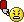Si si c'est bien l'url officielle : Support MS

A lire, le symptôme et les workarounds ... le 2 en particulier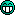Bon temps de midi sur ce !

##### Share on other sites

• 4 weeks later...

yop !

J'aimerais bien me mettre au c#, quelqu'un a une bonne référence de bouquin ou de site web à la limite ?

Merci bien !

##### Share on other sites

Il y en a plusieurs, à choisir en fonction de tes connaissances préalables en programmation. Quel est ton niveau sur d'autres éventuelles technologies ?

##### Share on other sites

disons que je me débrouille en programmation, je viens de terminer des études là dedans ^^ avec pour l'instant, plutôt C, java et python

##### Share on other sites

Bon alors ça fait longtemps que j'ai pas regardé de tutos mais tu trouvera une bonne liste d'éléments pas trop anciens ici. Il s'agit principalement de C# 2.0 qui est un bon point de départ pour évoluer ensuite vers 3.0-3.5 quand tu seras plus à l'aise.

##### Share on other sites

ok, je vais jeter un oeil à ça !

Merci !

##### Share on other sites

• 1 month later...

Pour ceux que ça pourrait intéresser,

Un article intéressant a été publié sur une équipe de chercheurs australiens qui a développé un micro kernel entièrement vérifié par la preuve formelle (7500 lignes de C). J'espère que ça pourra faire avancer cette discipline pour enfin pouvoir l'utiliser de manière automatique sur nos productions :)

##### Share on other sites

• 1 month later...

C´est cool la programmation.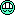Peu d´interventions, dommage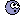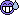##### Share on other sites

• 3 weeks later...

bonjour a tousje cherche ou demande un tuto sur la création de navigateur web développé en C ou C++

j'en ais trouvé un sur le site du zero mais il y a des problemes de dll donc je m'adresse a vous pour me montré la voix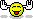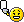si vous en avez un ou que vous avez les capacités a faire un tuto du ce genre je suis preneur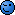merci de votre aide##### Share on other sites

On a peut-être les capacités de faire un tuto complet... Mais peut-être pas le temps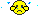(enfin je parle pour moi hein)

Si le tutoriel du site du rézo ne te convient pas, on peut soit essayer de voir ce qui ne fonctionne pas dedans, soit te donner des pistes sur des choses plus génériques à lire pour progresser...##### Share on other sites

se serais gentil de pouvoir m'aider oui

les probleme sont les DLL

elles donnent des erreurs du genre "Le point d'entrée de procédure

?insert@?\$QVector@VQpointF@@@@QAEXHABVQPointF@@@Z est introuvable dans la bibliothèque de lien dynamiques QtCore4.dll."

voila ce qui me donne ^^

alors quelqu'un sait?

## Join the conversation

You can post now and register later. If you have an account, sign in now to post with your account.×   Pasted as rich text.   Paste as plain text instead

Only 75 emoji are allowed.